Math-Tests.com# Algebra Worksheet

Note: You can use this algebra worksheet for educational noncommercial purposes only.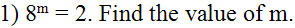2) What is the equation of this line?Answer: _______________________

3) Solve this absolute value inequality: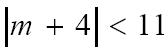6) What are the solutions of the equation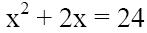7) This is the graph of which quadratic function?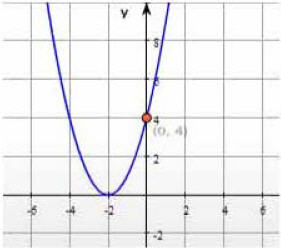Answer: ________________________

8) A rectangle has a perimeter of 22 inches and an area of 24 square inches. Find the length and the width of the rectangle.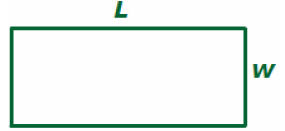Answer: ________________________

9) What inequality is graphed below?Answer: _________________________

10) Solve this proportion: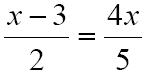Answer: ________________________

This Algebra Worksheet is provided by Math-Tests.com